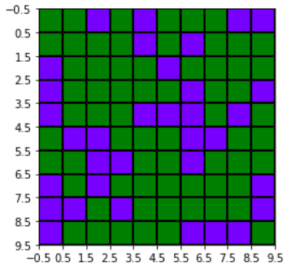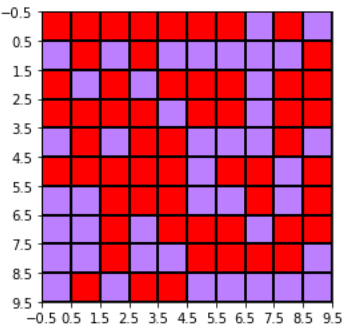Related Articles
Matplotlib.colors.to_hex() in Python
• Last Updated : 21 Apr, 2020

Matplotlib is an amazing visualization library in Python for 2D plots of arrays. Matplotlib is a multi-platform data visualization library built on NumPy arrays and designed to work with the broader SciPy stack.

## matplotlib.colors.to_hex()

The `matplotlib.colors.to_hex()` function is used to convert numbers between 0 to 1 into hex color code. It uses the `#rrggbb` format if keep_alpha is set to False(its also the default) else it uses `#rrggbbaa`.

Syntax: matplotlib.colors.to_hex(c, keep_alpha=False)

Parameters:

1. c: This represents an array of color sequence between 0 to 1.
2. keep_alpha: If set True uses #rrggbbaa format else uses #rrggbb format and it only accepts boolean values.

Example 1:

 `import` `matplotlib.pyplot as plt``from` `matplotlib ``import` `colors``import` `numpy as np`` ` `# dummy data to build the grid``data ``=` `np.random.rand(``10``, ``10``) ``*` `20`` ` `# converting into hex color code``hex_color``=``matplotlib.colors.to_hex([ ``0.47``, ``                                    ``0.0``, ``                                    ``1.0` `])`` ` `# create discrete colormap``cmap ``=` `colors.ListedColormap([hex_color, ``                              ``'green'``])`` ` `bounds ``=` `[``0``,``10``,``20``]``norm ``=` `colors.BoundaryNorm(bounds, cmap.N)`` ` `fig, ax ``=` `plt.subplots()``ax.imshow(data, cmap``=``cmap, norm``=``norm)`` ` `# draw gridlines``ax.grid(which``=``'major'``, axis``=``'both'``, ``        ``linestyle``=``'-'``, color``=``'k'``,``        ``linewidth``=``2``)`` ` `ax.set_xticks(np.arange(``-``.``5``, ``10``, ``1``));``ax.set_yticks(np.arange(``-``.``5``, ``10``, ``1``));`` ` `plt.show()`

Output:Example 2:

 `import` `matplotlib.pyplot as plt``from` `matplotlib ``import` `colors``import` `numpy as np`` ` `# dummy data to build the grid``data ``=` `np.random.rand(``10``, ``10``) ``*` `20`` ` `# converting into hex color``# code with alpha set to True``hex_color ``=` `matplotlib.colors.to_hex([ ``0.47``,``                                      ``0.0``, ``                                      ``1.0``, ``                                      ``0.5` `],``                                     ``keep_alpha ``=` `True``)`` ` `# create discrete colormap``cmap ``=` `colors.ListedColormap([hex_color, ``                              ``'red'``])`` ` `bounds ``=` `[``0``, ``10``, ``20``]``norm ``=` `colors.BoundaryNorm(bounds, cmap.N)`` ` `fig, ax ``=` `plt.subplots()``ax.imshow(data, cmap ``=` `cmap, norm ``=` `norm)`` ` `# draw gridlines``ax.grid(which ``=``'major'``, axis ``=``'both'``, ``        ``linestyle ``=``'-'``, color ``=``'k'``, ``        ``linewidth ``=` `2``)`` ` `ax.set_xticks(np.arange(``-``.``5``, ``10``, ``1``));``ax.set_yticks(np.arange(``-``.``5``, ``10``, ``1``));`` ` `plt.show()`

Output:Attention geek! Strengthen your foundations with the Python Programming Foundation Course and learn the basics.

To begin with, your interview preparations Enhance your Data Structures concepts with the Python DS Course.

My Personal Notes arrow_drop_up